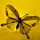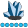## Run same procedure over multiple datasets and merge the results

Hi everyone!

I have about 93 individual datasets.  All these datasets have same column names. For each dataset, I will calculate a % based on one column "test_result".  The test result is coded as 1 (pass) and 0 (fail).

I use proc means sum to count total tests and number of pass and eventually get a % of the pass and output the % in a new dataset with a unique ID (linked back to the original dataset).

I need to run the same procedure for the 93 datasets, and make a new dataset to only have the all unique ID and % in.

I'm not familiar with macro and tried a few but did not seem work.

Any helps are appreciated!

5 REPLIES 5whymath
Lapis Lazuli | Level 10

## Re: Run same procedure over multiple datasets and merge the results

Use dictionary dataset and I/O function, you can do it in an easy way.

``````*Generate datasets for test;
data have1 have2;
call streaminit(42);
do i=1 to 42;
test_result=rand('binomial',0.5,1);
output have1;
end;
do i=1 to 6174;
test_result=rand('binomial',0.5,1);
output have2;
end;
run;

*Get count of 'test_result=1' with the help of dictionary dataset and I/O function;
data want;
set sashelp.vtable;
where libname='WORK' and memtype='DATA' and memname like 'HAVE%';

did=open(catx('.',libname,memname)||'(where=(test_result=1))');
if did then do;
nobs_1=attrn(did,'nlobsf');
did=close(did);
end;
percent=nobs_1/nlobs*100;

format percent 8.2;
keep libname memname memlabel nlobs nobs_1 percent;
run;``````whymath
Lapis Lazuli | Level 10

## Re: Run same procedure over multiple datasets and merge the results

If you are not familiar with dictionary dataset and I/O function, you can use proc freq to make it, too.

``````data mid1;
set have:(keep=test_result) indsname=indsname;
dsname=indsname;
run;

proc freq data=mid1 noprint;
tables test_result*dsname/out=want(where=(test_result=1) keep=dsname test_result pct_col) outpct;
run;``````

Now, you can check the dataset work.want.

## Re: Run same procedure over multiple datasets and merge the results

Create a DATA step view to concatenate all datasets, and use the INDSNAME= option to create a variable containing the dataset name. Then run your analysis BY this new variable in a single step.

## Re: Run same procedure over multiple datasets and merge the results

Your requirement of "only have the all unique ID and % " asks for something related to variable(?) named Id but is not described in your proc means or elsewhere in the description.

Which means that "unique ID and %" brings up a very significant question of what is the denominator to be used in the %? The occurences of ID across all the data sets? Within each data set?

A couple of small data sets as an example of input and the relatively easy to calculate by hand to show the example output for those would go a long way to clarification.

Here is an example of how to share data or provide examples with data step code:

```data d1;
input id \$  test_result;
datalines;
1   1
1   0
2   1
2   1
2   0
3   0
;
data d2;
input id \$  test_result;
datalines;
1   0
2   1
2   1
2   1
;
data d3;
input id \$  test_result;
datalines;
1   1
1   1
2   0
2   0
2   0
3   1
;```

Pasting the code into a text box opened on the forum with the </> icon above the message window sets it apart from general question text.

Note the above example specifically includes at least one Id not in each set. The example is small enough that you should be able to calculate whatever your result is by hand and show that, also best as data step code similar to shown.

If your data is significantly different in behavior than that shown you now have a pattern on how to provide such.

## Re: Run same procedure over multiple datasets and merge the results

It's easier to combine all datasets into one, adding in a variable that will uniquely identify each dataset and then do the calculation using the BY/CLASS feature of PROC MEANS.
Discussion stats
• 5 replies
• 226 views
• 2 likes
• 5 in conversation